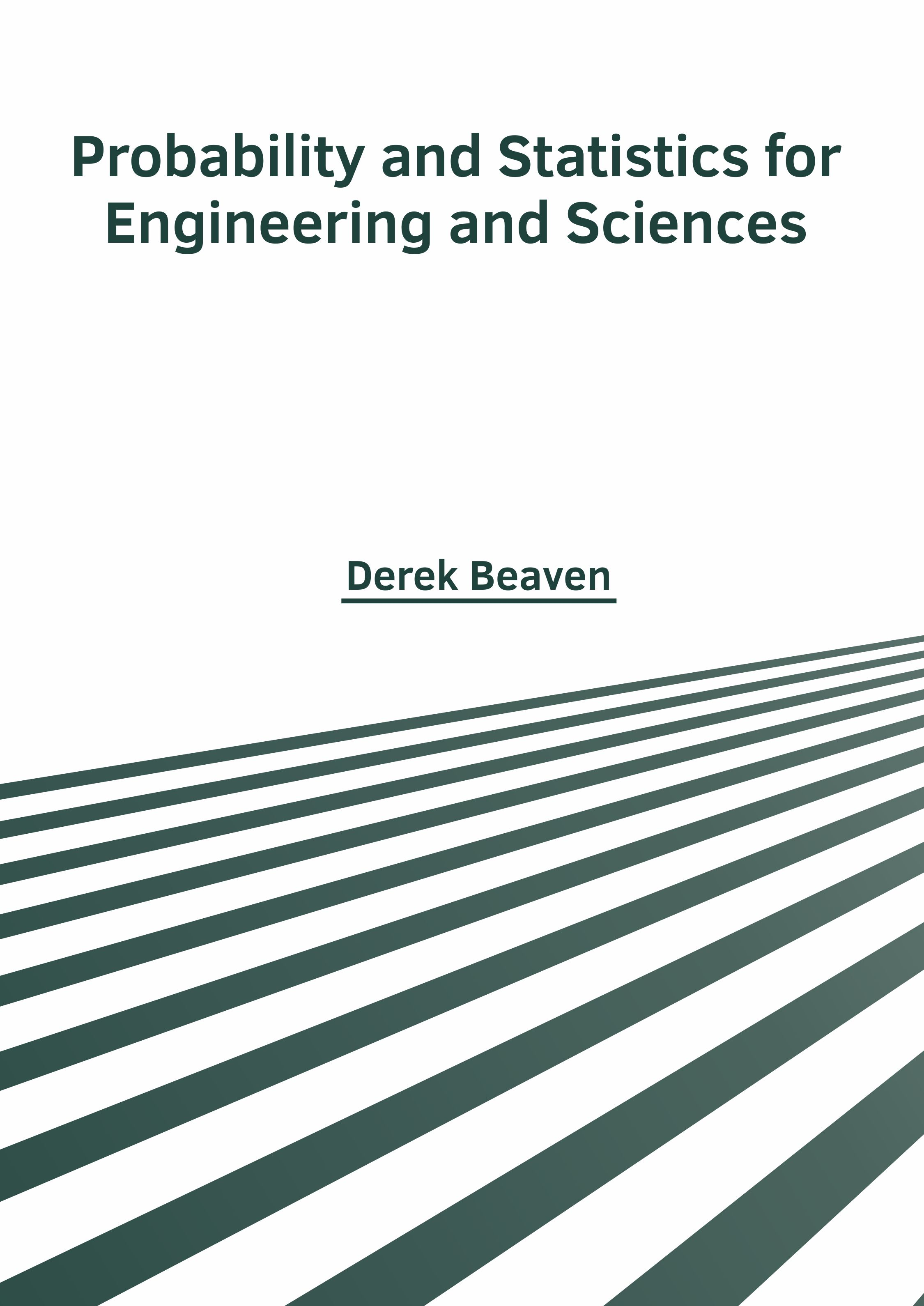BROWSE BY

#### Probability and Statistics for Engineering and Sciences9781632388803

Author

Derek Beaven

##### Publisher

NY Research Press

English

2022

##### Category

Mathematics - Probability and Statistics

241

\$ 160

##### Description

Probability and Statistics are two closely related sub-disciplines of mathematical. Statistics is a mathematical branch that deals with data collection, organization, interpretation, presentation and analysis. There are two main statistical methods used in data analysis - descriptive statistics and inferential statistics. Descriptive statistics summarize the data from a sample by using indexes like mean and standard deviation, whereas, inferential statistics concludes data that is subject to random variations. Probability is a measure that quantifies the likelihood that events are going to occur. It measures the quantity as a number between 0 and 1 that respectively indicate the impossibility and certainty of an event. Probability distributions are commonly used for statistical analysis. Both these topics are often studied in conjunction with one another. This book presents researches and studies performed by experts across the globe. It studies, analyses and upholds the pillars of probability and statistics and their utmost significance in modern times. This book attempts to assist those with a goal of delving into these areas.